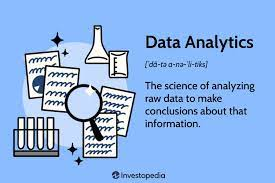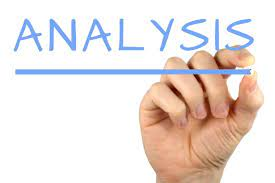Microsoft Excel is a popular software for managing data, with a user-friendly interface that makes data entry and analysis easy. Excel can be used to create simple spreadsheets or elaborate databases, and can be used to visualize data in a variety of ways. Excel can also be used to create financial models, create graphs and charts, and more.

Uses

Microsoft Excel is commonly used for tasks such as data entry, budgeting, and forecasting. It can also be used to create charts and graphs.

Microsoft excel tools

There are many ways you can use Excel to streamline your work. Here are a few examples:

1. Use Excel to keep track of your expenses. Create a spreadsheet with columns for each category of expense, and track how much you spend in each category each month. This will help you stay accountable and keep track of your spending.

2. Use Excel to track your progress on a project. Create a spreadsheet with columns for each step of the project, and track how much progress you have made in each step. This will help you stay on track and avoid getting bogged down in details.

4. Use Excel to create graphs and charts. Create graphs and charts to visualize data in your spreadsheet. This will help you see trends

Excel functions

Microsoft Excel is a powerful spreadsheet application that can be used for a variety of tasks, including data management, analysis, and presentation. Here are some of the most commonly used Excel functions:

Function Description

AVERAGE() The AVERAGE function averages the values in a column.

COUNT() The COUNT function counts the number of occurrences of a value in a column.

MAX() The MAX function returns the maximum value in a column.

MIN() The MIN function returns the minimum value in a column.

SUM() The SUM function calculates the sum of all values in a column.

var() the var function returns the value of a column that is a function of another column.

EXCEL DATA ANALYSIS

When it comes to data analysis, Microsoft Excel is a powerful tool that can be used to analyze a variety of data. One of the benefits of using Excel is that it is available on a wide range of platforms, making it easy to use. Additionally, Excel is free to download and use, making it an affordable option for data analysis.

One of the most common uses of Excel for data analysis is to summarize data. This can be done by creating charts and graphs, or by simply presenting the data in a summarized form. Additionally, Excel can be used to analyze data in more detail. For example, Excel can be used to calculate averages, to identify trends, and to perform mathematical operations on data.

Overall, Excel is a versatile tool that can be used for a variety of data analysis tasks. If you are looking for a cost-effective option for data analysis, Excel is a good option to consider.

Conditional formatting

In Microsoft Excel, conditional formatting is a formatting option that lets you apply different formatting to cells based on certain conditions. For example, you can use conditional formatting to make cells blue if they contain a value greater than 100, or to make cells red if they contain a value greater than 5000.

Conditional formatting is a great way to make your data easier to read and understand. By applying different formats to different cells, you can make it easy for you to see which cells contain important information, and which cells you should focus on.

To use conditional formatting in Microsoft Excel, first select the cells you want to format. Next, click the conditional formatting button (the one with the three lines in it) on the Home tab.

You’ll then be able to select the condition you want to apply to the cells. For example, you can use the drop-down list to choose whether the cells should be formatted blue if the value is greater than 100, or red if the value is less than 100. #MMBA3## AWOL & Ajaokuta Steel Mill – April 21 to 23.

in